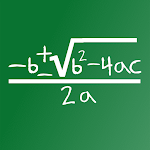• Author:

• Version:

1.2

• Update on:

## The description of Quadratic Equation Calculator/Math Equation

Quadratic calculator that shows the equation step by step, the rational and the irrational numbers, everything!

Mathematic calculator for the quadratic function (polynomial function, 2nd grade equation). Trinomial Equation.

Resolve in a very easy and simple way any quadratic equation. Just put \"a\", \"b\", and \"c\" and click solve. Really easy! just one button! Get both roots with this math calculator for quadratic functions.

This polynomial calculator also works when the equation has only one root or even none!

100% Tested and trustworthy, do not fail math again. Use this calculator of trinomial equations! Exceptional for math test and exams.

PUSH THE SOLVE RESULTS BUTTON TO SHOW THE IRRATIONAL ANSWERS!